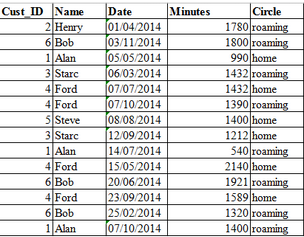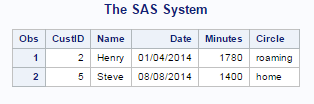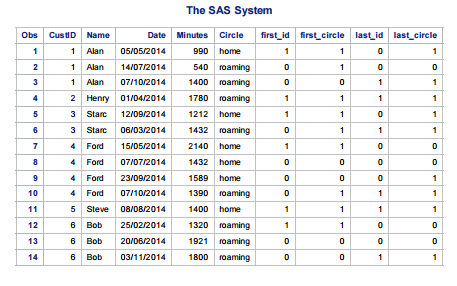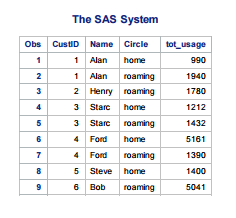Home » Effective data exploration / processing using FIRST. & LAST. in SAS PDV

# Effective data exploration / processing using FIRST. & LAST. in SAS PDV

Efficiency in coding differentiates a good coder from a bad coder. While you don’t need to be an awesome coder necessarily to be a good analyst, being a good coder always gives you that extra edge. In this article, I am explaining a few simple tricks with FIRST. & LAST. variables in SAS to help you become better in processing data.

### What is FIRST. & LAST. ?

The SET and BY statements in a data step tell SAS to process the data by grouping observations together. Whenever we use BY statement with a SET statement, SAS automatically creates two temporary variables for each variable name that appears in the BY statement. One of the temporary variables is called FIRST.variable, where variable is the variable name appearing in the BY statement. The other temporary variable is called LAST.variable. The two variables always equals either 1 or 0 when:

• FIRST.variable = 1 when an observation is the first observation in a BY group
• FIRST.variable = 0 when an observation is not the first observation in a BY group
• LAST.variable = 1 when an observation is the last observation in a BY group
• LAST.variable = 0 when an observation is not the last observation in a BY group

SAS uses the value of the FIRST.variable and LAST.variable to identify the first and last observations in a group. SAS places FIRST.variable and LAST.variable in the Program Data Vector (PDV). Then, they become available for DATA step processing but SAS does not add them to the output data set as they are temporary in nature.

### What are the uses of FIRST. and LAST. ?

Let’s look at some of the many uses of FIRST.variable and LAST.variable in SAS using the business problem below:

We have got a data set as shown below from a mobile network provider. It contains the following information:

• Customer ID
• Name of the Customer
• Date of Call
• Duration / Minutes used
• Circle – Home /RoamingWe need to perform the following exercises:

1. Find the total customers having only single day of usage.
2. Find the total usage of each customer in each of the circles – home & roaming circles.

Note: These problems form a very important part of the exploratory phase of the project and can provide key insights about your customers.

Can you think of ways to solve this problem? How easy or difficult does it look? Let’s solve them and see!

#### Problem 1: Find the total customers having only a single day of usage.

Answer: Since this dataset is small, we can easily make out ‘Henry’ and ‘Steve’ have only one record each in this dataset. Let’s try to find this answer using the concept of FIRST. and LAST. We’ll sort the data by Cust_ID then FIRST.Cust_ID and LAST.Cust_ID and make sure that these two records must be equal to one.

Lets verify this, by running the code below and observing the output:

Code:

```proc sort data = MinutesUsage;
by Cust_ID;
run;```
```data single;
set MinutesUsage;
by Cust_ID;
/* If the condition is satisfied we store the output in a dataset called single*/
if first.Cust_ID= 1 and last.Cust_ID = 1 then output single;
run;```

Output:#### Problem 2: Find the total usage of each customer for Home & Roaming Circles.

Answer: To solve this problem, I have first sorted the data by Cust_ID and then by Circle. Let’s see how the variables first. and last. are created for each BY variable:

Code:

```proc sort data = MinutesUsage;
by Cust_ID Circle;
run;

data details;
set MinutesUsage;
by Cust_ID Circle;

/* For each customer,the first record will have first.Cust_ID = 1*/
if first.Cust_ID = 1 then first_id = 1;else first_id = 0;

/* For each Customer,the first occurrence of a particular circle will have first.Circle = 1*/
if first.Circle = 1 then first_circle = 1;else first_circle = 0;

/* For each customer the last occurrence will have last.Cust_ID = 1*/
if last.Cust_ID = 1 then last_id = 1;else last_id = 0;

/* For each customer,the last occurrence of a particular circle will have last.Circle = 1*/
if last.Circle = 1 then last_circle = 1;else last_circle = 0;
run;```

Intermediate data set:Let’s understand the intermediate data set shown above by focussing at customer “Alan”:

• The sorting is done first by Cust_ID and then by Circle, hence first_id and first_circle should be 1 for the first record of Alan.
• It is also the first and last record for circle “home”, hence the first_circle and last_circle equal to 1.
• The second record of Alan is the first occurrence of Circle “roaming, hence first_circle equals to 1
• Next one is the last record for Alan, last_id = 1  and hence the last_circle also equals to 1

Let’s look at the below codes to evaluate the total usage by customer and circle:

Code:

```proc sort data = MinutesUsage;
by Cust_ID Circle;
run;

data tot_usage (keep = Cust_ID name Circle tot_usage);
set MinutesUsage;
by Cust_ID Circle;
/* Step 1*/
if first.Circle = 1 then tot_usage = 0;
/* Step 2*/
tot_usage + minutes;
/*Step 3*/
if last.circle = 1 then output;
run;```

Let’s understand the code by looking at the intermediate data set:

1. For Alan,the first record is for home circle and thus first. circle is 1. We initialize tot_usage = 0 in Step 1, then we add minutes to tot_usage in Step 2.
2. The same record is also the last record of home circle for Alan. So for last. circle = 1, we just add the variable tot_usage to the output dataset tot_usage in Step 3.
3. For Alan, the second record is the first occurrence of circle = roaming, so Step 1 – 2 is repeated. The value of tot_usage now is 540.
4. In Step 3,the value of last. circle is not 1 and thus the value of tot_usage remains 540.
5. For the third record, first. circle is not = 0, thus only Step 2 is executed. Now tot_usage is 1940 and it is also the record for which last. circle = 1 and hence the variable tot_usage is added to the output dataset tot_usage.
6. All these steps are repeated for each Cust_ID and Circle group and the tot_usage dataset is created.

Output:### End Notes:

In this article, we discussed the concept of FIRST. and LAST. in SAS using an example. If you have used it in any other way, or if there is a better way of implementing this concept, please do make suggestions.This article has been contributed by Shuvayan Das. Shuvayan has 4 years of experience with TCS, has undergone training with Jigsaw Academy and is proficient with SAS, SQL & Excel. He was also featured as Newbie of the month in our recent newsletter and happens to be one of the most active users on Analytics Vidhya Discuss.

### If you like what you just read & want to continue your analytics learning, subscribe to our emails, follow us on twitter or like our facebook page.

You can also read this article on our Mobile APP•Vishu says:

very nice post about first. and .last.

This is best example to understand

Thank you

•Swapnika says:

Here, while calculating the tot_usage, we have to “retain” this variable right?
As sas statements are computed for each row in the dataset

•kranthi kotturi says:

clear and perfect example to understand First. and Last.

•Anupam says:

I have a dataset for a test: (date is in mmddyy10. format, test result is in binary format 0 for negative and 1 for positive)
ID date test_result
1 06/05/2016 0
1 06/06/2016 0
1 06/07/2016 1
1 06/08/2016 0
1 06/08/2016 1
2 08/05/2016 0
2 09/06/2016 1
2 10/07/2016 1
2 11/08/2016 0
2 12/08/2016 1

I am interested in all observations for every ID until first positive test (first 3 obs for ID1 and first 2 for ID2).
I want to create this data as a subset; how do I do it using first. function?

•Suman Kumar says:

U really makes so easy to understand ..really nice